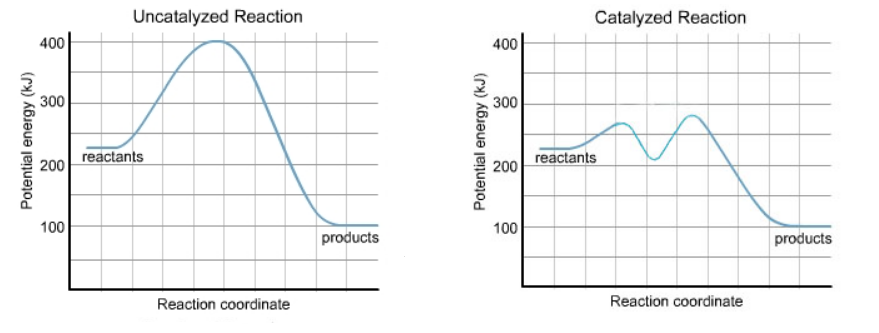# Problem: Part AWhat is the value of the activation energy of the uncatalyzed reaction?Express your answer to three significant figures and include the appropriate units.Part BWhat is the value of the enthalpy change of the uncatalyzed reaction?Express your answer to three significant figures and include the appropriate units.Part CWhat is the value of the activation energy of the uncatalyzed reaction in reverse?Part DWhat is the value of the enthalpy change of the uncatalyzed reaction in reverse?Express your answer to three significant figures and include the appropriate units.

###### FREE Expert Solution
80% (208 ratings)
###### FREE Expert Solution
80% (208 ratings)###### Problem Details

Part A

What is the value of the activation energy of the uncatalyzed reaction?

Express your answer to three significant figures and include the appropriate units.

Part B

What is the value of the enthalpy change of the uncatalyzed reaction?

Express your answer to three significant figures and include the appropriate units.

Part C

What is the value of the activation energy of the uncatalyzed reaction in reverse?

Part D

What is the value of the enthalpy change of the uncatalyzed reaction in reverse?

Express your answer to three significant figures and include the appropriate units.What scientific concept do you need to know in order to solve this problem?

Our tutors have indicated that to solve this problem you will need to apply the Energy Diagram concept. If you need more Energy Diagram practice, you can also practice Energy Diagram practice problems.

What is the difficulty of this problem?

Our tutors rated the difficulty ofPart AWhat is the value of the activation energy of the unca...as medium difficulty.

How long does this problem take to solve?

Our expert Chemistry tutor, Dasha took 6 minutes and 58 seconds to solve this problem. You can follow their steps in the video explanation above.

What professor is this problem relevant for?

Based on our data, we think this problem is relevant for Professor Johnson's class at GS.